/

### The negative binomial distribution

The negative binomial distribution describes the probability of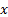successes in a sequence of independent experiments each with likelihood of success of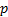that arise before there arefailures. In this interpretationis a positive integer, but the distributional definition can also be extended to real values of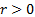. Note: different texts adopt slightly different definitions, e.g. with support starting at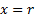not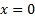and/or withdenoting probability of failure rather than probability of success.

 Distribution name Negative binomial distribution Common notation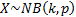Parameters= number of failures ()= probability of success in each experiment (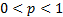) Support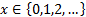Probability mass function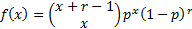Ifis non-integral then is: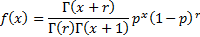Cumulative distribution function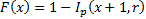Mean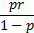Variance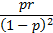Skewness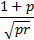(Excess) kurtosis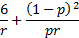Characteristic function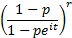Other comments The geometric distribution is the same as the negative binomial distribution with parameter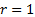. Its pdf and cdf are therefore: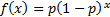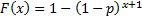For the special case whereis an integer the negative binomial distribution is also called the Pascal distribution. The Poisson distribution is also a limiting case of the negative binomial: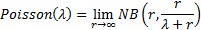Nematrian web functions

Functions relating to the above distribution may be accessed via the Nematrian web function library by using a DistributionName of “negative binomial”. For details of other supported probability distributions see here.